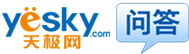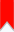#75分最佳答案
2013-8-4 18:50
•小魏703
•1.Tn=9.55*Pn/Nn=591.5N.M 2.Rf=Un/Ifn=440/5=88欧姆 Pem=Pn+In^2*Ra+Ifn^2*Rf=96000+255^2*0.078+5^2*88=103271.95W Tem=9.55*Pem/Nn=636.3N.M 3.Ce*Fain=(Un-In*Ra)/Nn=0.27 n=Un/Ce*Fain=1630r/min
• 2
对我很有用
• 提问者谁又是谁的谁谁谁的感言：谢谢您的解答！